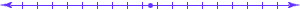## Computer Science 456/656Automata and Formal Languages## Why do we introduce the concept "language" in a course about the theory of computation?

The answer is simple and striking:

Every problem that has a mathematical definition is a language problem.

The above statements seems quite radical, but it is simple to explain by giving a few examples.

Let L1 be the language of all non-empty strings of decimal digits.
Let L2 be the language of all two term addition problems, that is, L2 consists of all strings of the form x+y, where x and y are in L1.
The function which we call "addition" has domain L2 and range L1, and, for example, takes the string 34+689 to the string 723.
The problem of "adding" is then the problem of computing this function.
Existence of a Cycle in a Directed Graph:
Consider the following problem. Given a directed graph G, does G contain a cycle?
We can encode G as a string. (Think: if you were to write a computer program to solve this problem, you would take as input some sort of file which encodes G. This file is in fact a string of symbols.)
We can then define L1 to be the language of all encodings of directed graphs, and L2 to be the language of all encodings of cyclic directed graphs.
Our problem is then, given a string w in L1, is w in L2?